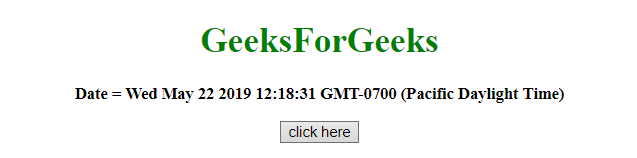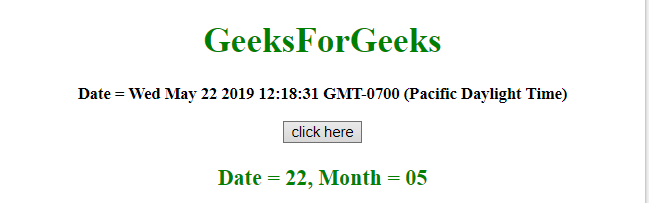# How to get Month and Date of JavaScript in two digit format ?

Given a date and the task is to get the Month and Date in 2 digit format. Use JavaScript methods to get the Month and Date in 2 digit format.

• JavaScript getDate() Method: This method returns the day of the month (from 1 to 31) for the defined date.

Syntax:

`Date.getDate()`

Return value: It returns a number, from 1 to 31, representing the day of the month.

• JavaScript getMonth() Method: This method returns the month (from 0 to 11) for the defined date, based on to local time.

Syntax:

`Date.getMonth()`

Return value: It returns a number, from 0 to 11, representing the month.

• JavaScript String slice() method: This method gets part of a string and returns the extracted parts in a new string. It uses the start and end parameters to define the part of the string to extract. First character starts from position 0, the second has position 1, and so on.

Syntax:

`string.slice(start, end)`

Parameters:

• start: This parameter is required. It specifies the position from where to start the extraction. First character is at position 0
• end: This parameter is optional. It specifies the position (excluding it) where to stop the extraction. If not used, slice() selects all characters from the start-position till the end of string.

Return value: It returns a string, representing the extracted part of the string.

Example 1: This example first get the Date and Month and then slicing properly to get them in 2 digit format by using getDate(), getMonth() and slice() method.

 `  ` `<``html``>  ` `    ``<``head``>  ` `        ``<``title``>  ` `            ``How to get the Month and Date of  ` `            ``JavaScript in 2 digit format ` `        `` ` `    ``  ` `     `  `    ``<``body` `style` `= ``"text-align:center;"``> ` `          `  `        ``<``h1` `style` `= ``"color:green;"` `>  ` `            ``GeeksForGeeks  ` `        `` ` `         `  `        ``<``p` `id` `= ``"GFG_UP"` `style` `= ``"font-size: 15px; font-weight: bold;"``> ` `        `` ` `         `  `        ``<``button` `onclick` `= ``"gfg_Run()"``>  ` `            ``click here ` `        `` ` `         `  `        ``<``p` `id` `= ``"GFG_DOWN"` `style` `=  ` `            ``"color:green; font-size: 20px; font-weight: bold;"``> ` `        `` ` `         `  `        ``<``script``> ` `            ``var el_up = document.getElementById("GFG_UP"); ` `            ``var el_down = document.getElementById("GFG_DOWN"); ` `            ``var today = new Date(); ` `            ``el_up.innerHTML = "Date = " + today; ` `             `  `            ``function gfg_Run() { ` `                ``var a = new Date(); ` `                ``var month = ("0" + (a.getMonth() + 1)).slice(-2); ` `                ``var date = ("0" + a.getDate()).slice(-2); ` `                ``el_down.innerHTML = "Date = " + date + ", Month = " + month; ` `            ``}          ` `        ``  ` `    ``  ` `                     `

Output:

• Before clicking on the button:• After clicking on the button:Example 2: This example creates a function which adds zero to the Date if it is less than 10 and adds zeros appropriately for the Month also, by using getDate() and getMonth() method.

 `  ` `<``html``>  ` `    ``<``head``>  ` `        ``<``title``>  ` `            ``How to get the Month and Date of ` `            ``JavaScript in 2 digit format ` `        `` ` `    ``  ` `     `  `    ``<``body` `style` `= ``"text-align:center;"``> ` `          `  `        ``<``h1` `style` `= ``"color:green;"` `>  ` `            ``GeeksForGeeks  ` `        `` ` `         `  `        ``<``p` `id` `= ``"GFG_UP"` `style` `= ``"font-size: 15px; font-weight: bold;"``> ` `        `` ` `         `  `        ``<``button` `onclick` `= ``"gfg_Run()"``>  ` `            ``click here ` `        `` ` `         `  `        ``<``p` `id` `= ``"GFG_DOWN"` `style` `=  ` `            ``"color:green; font-size: 20px; font-weight: bold;"``> ` `        `` ` `         `  `        ``<``script``> ` `            ``var el_up = document.getElementById("GFG_UP"); ` `            ``var el_down = document.getElementById("GFG_DOWN"); ` `            ``var today = new Date(); ` `            ``el_up.innerHTML = "Date = " + today; ` `             `  `            ``function formatDateToString(date) { ` `                ``var dd = (date.getDate() < ``10` `? '0' : '') ` `                        ``+ date.getDate(); ` `                         `  `                ``var MM = ((date.getMonth() + 1) < 10 ? '0' : '') ` `                        ``+ (date.getMonth() + 1); ` `                         `  `                ``return "``Date` `= ``" + dd + "``, Month = " + MM; ` `            ``} ` `             `  `            ``function gfg_Run() { ` `                ``var ``a` `= ``new` `Date(); ` `                ``el_down.innerHTML` `= ``formatDateToString``(a); ` `            ``}          ` `        ``  ` `    ``  ` `                     `

Output:

• Before clicking on the button:• After clicking on the button:My Personal Notes arrow_drop_upCheck out this Author's contributed articles.

If you like GeeksforGeeks and would like to contribute, you can also write an article using contribute.geeksforgeeks.org or mail your article to contribute@geeksforgeeks.org. See your article appearing on the GeeksforGeeks main page and help other Geeks.

Please Improve this article if you find anything incorrect by clicking on the "Improve Article" button below.